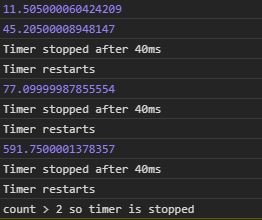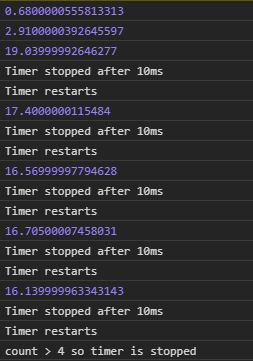# D3.js timer.restart() Function

The timer.restart() function in D3.js is used to restart a timer with the given function and delay. The timer.restart() function is used when one wants to reset the timer and start again.

Syntax:

```timer.restart(callback, delay);
```

Parameters: It takes two parameters as mentioned above and described below:

• callback: It is the function to be stopped or start after a particular delay.
• delay: It is the time after which the function will be executed or stop

Example 1: When no delay is given.

## HTML

 ` ` `<``html` `lang``=``"en"``> ` ` `  `<``head``> ` `    ``<``meta` `charset``=``"UTF-8"``> ` `    ``<``meta` `name``=``"viewport"` `content``= ` `        ``"width=device-width, initial-scale=1.0"``> ` ` ` ` `  `<``body``> ` `    ``` `    ``<``script` `type``=``"text/javascript"`  `        ``src``=``"https://d3js.org/d3.v4.min.js"``> ` `    `` ` `     `  `    ``<``script``> ` `        ``count = 0; ` `        ``let func = function (e) { ` `            ``console.log(e) ` `            ``if (e > 40) { ` `                ``console.log("Timer stopped after 40ms") ` `                ``if (e > 40) { ` `                    ``count++; ` ` `  `                    ``// Restarting the timer again ` `                    ``console.log("Timer restarts") ` `                    ``timer.restart(func) ` `                ``} ` `                ``if (count > 2) { ` `                    ``timer.stop(); ` `                    ``console.log( ` `                        ``"count > 2 so timer is stopped") ` `                ``} ` `            ``} ` `        ``} ` `        ``var timer = d3.timer(func); ` `    `` ` ` ` ` `  ``

Output:Example 2: When a delay is given.

## HTML

 ` ` `<``html` `lang``=``"en"``> ` ` `  `<``head``> ` `    ``<``meta` `charset``=``"UTF-8"``> ` `    ``<``meta` `name``=``"viewport"` `content``= ` `        ``"width=device-width, initial-scale=1.0"``> ` ` ` ` `  `<``body``> ` `    ``` `    ``<``script` `type``=``"text/javascript"`  `        ``src``=``"https://d3js.org/d3.v4.min.js"``> ` `    `` ` `     `  `    ``<``script``> ` `        ``count = 0; ` `        ``let func = function (e) { ` `            ``console.log(e) ` `            ``if (e > 10) { ` `                ``console.log("Timer stopped after 10ms") ` `                ``if (e > 10) { ` `                    ``count++; ` ` `  `                    ``// Restarting the timer again ` `                    ``console.log("Timer restarts") ` `                    ``timer.restart(func) ` `                ``} ` `                ``if (count > 4) { ` `                    ``timer.stop(); ` `                    ``console.log( ` `                    ``"count > 4 so timer is stopped") ` `                ``} ` `            ``} ` `        ``} ` `        ``// A delay of 2000ms ` `        ``var timer = d3.timer(func, 2000); ` `    `` ` ` ` ` `  ``

Output:My Personal Notes arrow_drop_upCheck out this Author's contributed articles.

If you like GeeksforGeeks and would like to contribute, you can also write an article using contribute.geeksforgeeks.org or mail your article to contribute@geeksforgeeks.org. See your article appearing on the GeeksforGeeks main page and help other Geeks.

Please Improve this article if you find anything incorrect by clicking on the "Improve Article" button below.

Article Tags :

Be the First to upvote.

Please write to us at contribute@geeksforgeeks.org to report any issue with the above content.